### Introduction to Euclids Geometry - Solutions

CBSE Class 9 Mathematics

NCERT Solutions
CHAPTER 5
Introduction to Euclids Geometry(Ex. 5.1)

1. Which of the following statements are true and which are false ? Give reasons for your answers.

(i) Only one line can pass through a single point.

(ii) There are infinite number of lines which pass through two distinct points.

(iii) A terminated line can be produced indefinitely on both the sides.

(iv) If two circles are equal, then their radii are equal.

(v) In Fig. 5.9, if AB = PQ and PQ = XY, then AB = XY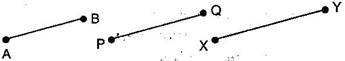Ans.(i) False

Correct statement: Infinite many lines can pass through a single point.

This is self-evident and can be seen visually by the student given below: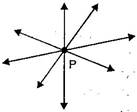(ii) False because the given statement contradicts the postulate I of the Euclid that assures that there is a unique line that passes through two distinct points.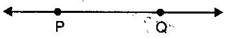Through two points P and Q a unique line can be drawn.

(iii) True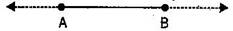Reason: We need to consider Euclid’s Postulate 2: “A terminated line can be produced indefinitely.”

(iv) True

Reason: Let us consider two circles with same radii.

We can conclude that, when we make the two circles overlap with each other, we will get a superimposed figure of the two circles.

Therefore, we can conclude that the radii of both the circles will also coincide and will be same.

(v) True

Reason: We are given that AB = PQ and PQ = XY.

By Euclid's axiom 1 i.e.,things which are equal to the same thing are equal to one another.

Therefore, we can conclude that AB, PQ and XY are the lines with same dimensions, and hence if AB = PQ and PQ = XY, then AB = XY.

2. Give a definition for each of the following terms. Are there other terms that need to be defined first? What are they, and how might you define them ?

(i) parallel lines

(ii) perpendicular lines

(iii) line segment

(v) square

Ans. (i) Parallel lines

Two lines are said to be parallel, when the perpendicular distance between these lines is always constant or we can say that the lines that never intersect each other are called as parallel lines.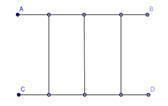We need to define line first, in order to define parallel lines.

(ii) Perpendicular lines

Two lines are said to be perpendicular lines, when angle between these two lines is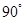.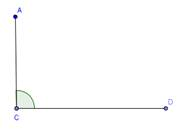We need to define line and angle, in order to define perpendicular lines.

(iii) Line segment

A line of a fixed dimension between two given points is called as a line segment.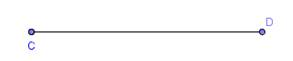We need to define line and point, in order to define a line segment.

The distance of any point lying on the boundary of a circle from the center of the circle is called as radius of a circle.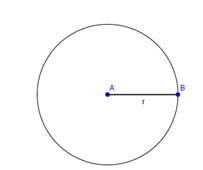We need to define circle and center of a circle, in order to define radius of a circle.

(v) Square

A quadrilateral with all four sides equal and all four angles ofis called as a square.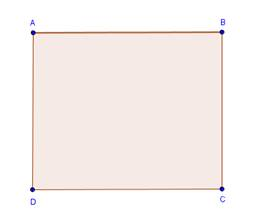We need to define quadrilateral and angle, in order to define a square.

3. Consider the two ‘postulates’ given below:

(i) Given any two distinct points A and B, there exists a third point C, which is between A and B.

(ii) There exists at least three points that are not on the same line.

Do these postulates contain any undefined terms ? Are these postulates consistent ? Do they follow from Euclid’s postulates ? Explain.

Ans. We are given with following two postulates

(i) Given any two distinct points A and B, there exists a third point C, which is between A and B.

(ii) There exists at least three points that are not on the same line.

The undefined terms in the given postulates are point and line.

The two given postulates are consistent, as they do not refer to similar situations and they refer to two different situations.

We can also conclude that, it is impossible to derive at any conclusion or any statement that contradicts any well-known axiom and postulate.

The two given postulates do not follow from the postulates given by Euclid.

The two given postulates can be observed following from the axiom, “Given two distinct points, there is a unique line that passes through them”.

4. If a point C lies between two points A and B such that AC = BC, then prove that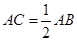. Explain by drawing the figure.

Ans. We are given that a point C lies between two points B and C, such that AC = BC.

We need to prove that.

Let us consider the given below figure.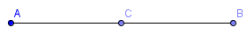We are given that AC = BC.…(i)

An axiom of the Euclid says that “If equals are added to equals, the wholes are equal.”

Let us add AC to both sides of equation (i).

AC AC = BC + AC.

An axiom of the Euclid says that “Things which coincide with one another are equal to one another.”

We can conclude that BC + AC coincide with AB, or

AB = BC + AC.…(ii)

An axiom of the Euclid says that “Things which are equal to the same thing are equal to one another.”

From equations (i) and (ii), we can conclude that

AC + AC = AB, or2AC = AB.

An axiom of the Euclid says that “Things which are halves of the same things are equal to one another.”

Therefore, we can conclude that.

5. In the above question, point C is called a mid-point of line segment AB, prove that every line segment has one and only one mid-point.

Ans. We need to prove that every line segment has one and only one mid-point.

Let us consider the given below line segment AB and assume that C and D are the mid-points of the line segment AB.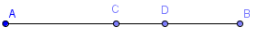If C is the mid-point of line segment AB, then

AC CB.

An axiom of the Euclid says that “If equals are added to equals, the wholes are equal.”

AC AC CB + AC.-----------(i)

From the figure, we can conclude that CB + AC will coincide with AB.

An axiom of the Euclid says that “Things which coincide with one another are equal to one another.”

AC AC AB.--------------------(ii)

An axiom of the Euclid says that “Things which are equal to the same thing are equal to one another.”

Let us compare equations (i) and (ii), to get

AC + AC = AB, or2AC = AB.----------------(iii)

If D is the mid-point of line segment AB, then

An axiom of the Euclid says that “If equals are added to equals, the wholes are equal.”

From the figure, we can conclude that DB + AD will coincide with AB.

An axiom of the Euclid says that “Things which coincide with one another are equal to one another.”

An axiom of the Euclid says that “Things which are equal to the same thing are equal to one another.”

Let us compare equations (iv) and (v), to get

An axiom of the Euclid says that “Things which are equal to the same thing are equal to one another.”

Let us compare equations (iii) and (vi), to get

An axiom of the Euclid says that “Things which are halves of the same things are equal to one another.”

Therefore, we can conclude that the assumption that we made previously is false and a line segment has one and only one mid-point.

6. In the following figure, if AC = BD, then prove that AB = CD.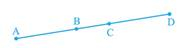Ans.
We are given that AC = BD.

We need to prove that AB = CD in the figure given below.From the figure, we can conclude that

AC = AB + BC, and

BD = CD + BC.

An axiom of the Euclid says that “Things which are equal to the same thing are equal to one another.”

AB + BC CD + BC. -------------(i)

An axiom of the Euclid says that “when equals are subtracted from equals, the remainders are also equal.”

We need to subtract BC from equation (i), to get

AB + BC - BC CD + BC - BC

AB = CD.

Therefore, we can conclude that the desired result is proved.

7. Why is axiom 5, in the list of Euclid’s axioms, considered as a ‘universal truth’? (Note that the question if not about fifth postulate)

Ans. We need to prove that Euclid’s fifth axiom is considered as a universal truth.

Euclid’s fifth axiom states that “the whole is greater than the part.”

The above given axiom is a universal truth. We can apply the fifth axiom not only mathematically but also universally in daily life.

Mathematical proof:

Let us consider a quantity z, which has different parts as abx and y.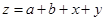.

Therefore, we can conclude that z will always be greater than its corresponding parts abx and y.

Universal proof:

We know that Mumbai is located in Maharashtra and Maharashtra is located in India.

In other words, we can conclude that Mumbai is a part of Maharashtra and Maharashtra is a part of India.

Therefore, we can conclude that whole India will be greater than Mumbai or Maharashtra or both.

Therefore, we can conclude that Euclid’s fifth axiom is considered as a ‘Universal truth’.